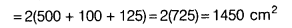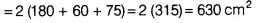# Shanti Sweets Stall was placing an order for making cardboard boxes for packing

Shanti Sweets Stall was placing an order for making cardboard boxes for packing their sweets. Two sizes of boxes were required. The bigger of dimensions 25 cm x 20 cm x 5 cm and the smaller of dimensions 15 cm x12 cm x 5 cm. For all the overlaps, 5% of the total surface area is required extra. If the cost of the cardboard is ₹4 for 1000 cm2, then find the cost of cardboard required for supplying 250 boxes of each kind.

Dimensions of bigger box are
l = 25 cm, b = 20 cm and h = 5 cm
∴ Total surface area of the bigger box
= 2(lb + bh + hl) = 2(25 x 20 + 20 x 5 + 5 x 25)Dimensions of smaller box are
l= 15 cm, b = 12 cm and h = 5 cm
∴ Total surface area of the smaller box
= 2(15 x 12 + 12 x 5 + 5 x 15)Total surface area of both boxes = Total surface area of bigger box + Total surface area of smaller box